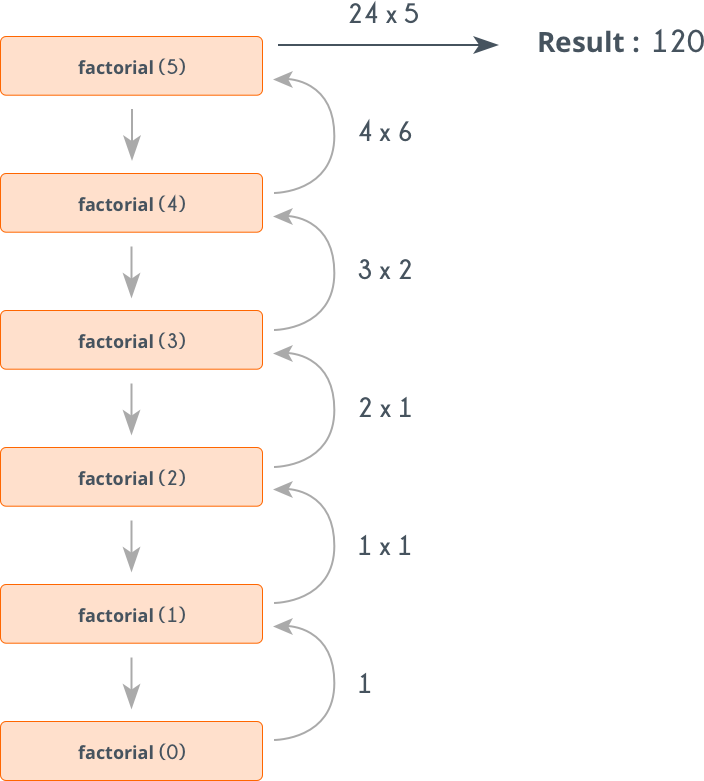# Factorialize a number - help understanding recursion

Tell us what’s happening:

So I’ve passed this activity and I deliberately attempted to pass it using recursion because it’s something I still am struggling to grasp. I think I understand it so far, but I’m hoping you can confirm my understanding.

First here is my code:

``````function factorialize(num) {
if (num <= 0) {
return 1;
} else {
return factorialize(num - 1) * num;
}

}

factorialize(5);
``````

Now my question:

I understand that if `factorialize(5)` is passed in it first stores 5. Then it subtracts 1 and stores 4. Then it subtracts 1 again and stores 3 and so on. Is that correct?

Thanks,

``````

User Agent is: <code>Mozilla/5.0 (Windows NT 10.0; Win64; x64) AppleWebKit/537.36 (KHTML, like Gecko) Chrome/84.0.4147.135 Safari/537.36</code>.

**Challenge:** Factorialize a Number

**Link to the challenge:**
https://www.freecodecamp.org/learn/javascript-algorithms-and-data-structures/basic-algorithm-scripting/factorialize-a-number``````

Basically that’s what happens. It needs a base case, in your case it’s `num <= 0`, so it does not infinite loop. Here’s a diagram to better explain:2 Likes

Thank you so much. Sometimes all I need is confirmation that my understanding is correct and you provided that.1 Like